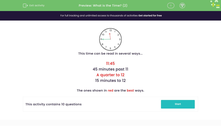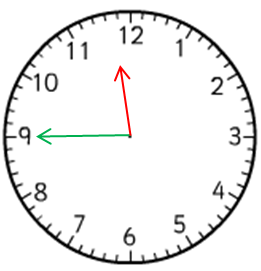# Tell the Time

In this worksheet, students will practise telling the time from an analogue clock face.Key stage:  KS 2

Curriculum topic:   Measurement

Curriculum subtopic:   Tell the Time (analogue, 12-hour clocks, 24-hour clocks)

Difficulty level:#### Worksheet Overview

In this activity, we will be learning to read the exact time on an analogue clock, using the position of the hands.

Clocks have an hour hand and a minute hand (and sometimes a second hand)

The hour hand is shorter than the minute hand.

Let's try an example question.

Example
What time is shown on this clock?This time can be read in several ways:

The hour hand is between the 11 and 12, but it is much closer to the 12.

So we know that the time is just before 12 o'clock.

The minute hand is pointing exactly at the 9.

Each number on the clock face represents 5 minutes, so the 9 represents 9 x 5 minutes, which is 45 minutes past the last hour or 15 minutes to the next hour.

There are 60 minutes in one hour and one-quarter of 60 is 15, so when the minute hand points to the 9, we can also say that the time is 'quarter to'.

The most common ways to write the time shown are:

11:45  or quarter to 12

You might also say:

15 minutes to 12 or 45 minutes past 11.

These would be correct, but less common ways of telling the time.

Now it's time to try some questions yourself!